Student Resources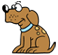Don't want

Big Ideas Math: Algebra 1

Chapter 1: Solving Linear Equations (pp. 1 - 40)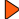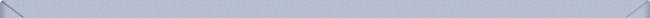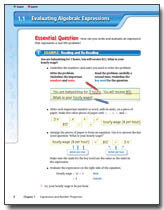Record and Practice Journal Color Manipulatives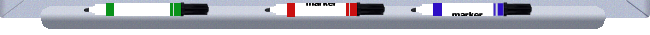Chapter 2: Graphing and Writing Linear Equations (pp. 41 - 102)Record and Practice Journal Color ManipulativesChapter 3: Solving Linear Inequalities (pp. 103 - 152)Record and Practice Journal Color ManipulativesChapter 4: Solving Systems of Linear Equations (pp. 153 - 200)Record and Practice Journal Color ManipulativesChapter 5: Linear Functions (pp. 201 - 258)Record and Practice Journal Color ManipulativesChapter 6: Exponential Equations and Functions (pp. 259 - 326)Record and Practice Journal Color ManipulativesChapter 7: Polynomial Equations and Factoring (pp. 327 - 400)Record and Practice Journal Color ManipulativesChapter 8: Graphing Quadratic Functions (pp. 401 - 452)Record and Practice Journal Color ManipulativesChapter 9: Solving Quadratic Equations (pp. 453 - 500)Record and Practice Journal Color ManipulativesChapter 10: Square Root Functions and Geometry (pp. 501 - 540)Record and Practice Journal Color ManipulativesChapter 11: Rational Equations and Functions (pp. 541 - 604)Record and Practice Journal Color ManipulativesChapter 12: Data Analysis and Displays (pp. 605 - 673)Record and Practice Journal Color Manipulatives